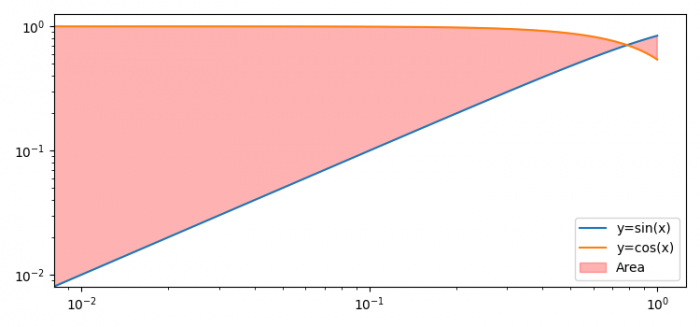# Fill the area under a curve in Matplotlib python on log scale

To fill the area under a curve in Matplotlib python on log scale, we can take the following steps−

• Set the figure size and adjust the padding between and around the subplots.
• Create x, y1 and y2 data points using numpy.
• Plot x, y1 and y2 data points using plot() method.
• Fill the area between the two curves.
• Set the scale of the axes.
• Place a legend on the plot.
• To display the figure, use show() method.

## Example

import numpy as np
from matplotlib import pyplot as plt

plt.rcParams["figure.figsize"] = [7.50, 3.50]
plt.rcParams["figure.autolayout"] = True

x = np.linspace(-1, 1, 100)
y1 = np.sin(x)
y2 = np.cos(x)

plt.plot(x, y1, label="y=sin(x)")
plt.plot(x, y2, label="y=cos(x)")

plt.fill_between(x, y1, y2, color="red", label="Area", alpha=0.3)

plt.xscale('log')
plt.yscale('log')

plt.legend(loc='lower right')

plt.show()

## Output